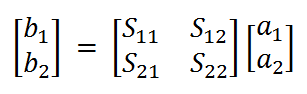#### Two-port network conversion tool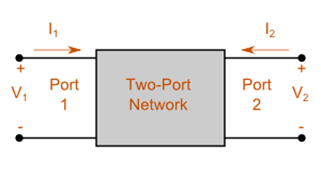An example of a general two-port network with voltage and currents defined.

This calculator allows the conversion between various two-port parameters. The two-port parameters include Z (impedance), Y (admittance), ABCD (chain) and S (scattering).

Most microwave textbooks [e.g. Pozar] provide very useful conversion formulas to switch between various two-port parameter conventions. Often only conversions for simplified cases are provided, namely where the impedance values of port 1 (source) and port 2 (load) are real, equal, or where a common port and system impedance is enforced.

This calculator allows for the impedance of the two ports to be complex and unique by using the equations developed by Frickey. The image below shows a standard two-port network. The basic two-port equations are shown thereafter.

Z-parameters: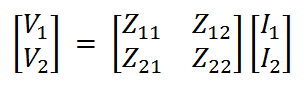Y-parameters: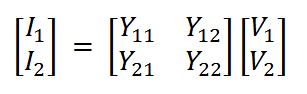ABCD-parameters: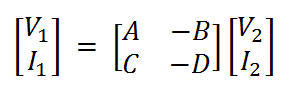S-parameters: